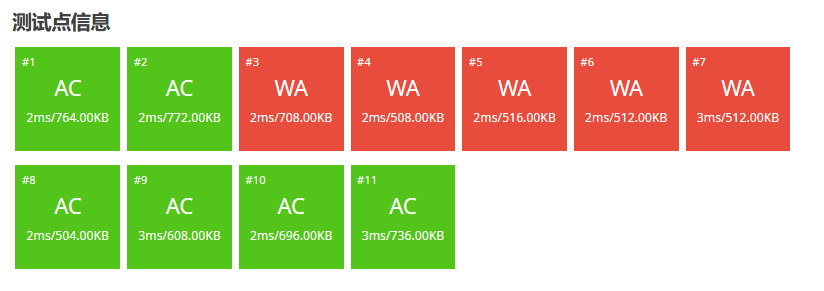# 每日洛谷_9.28

## 题目描述3       6

0       15

## 说明与提示

• 对于 50%50 %50% 的数据，保证 3≤n≤100。
• 对于 100%100 %100% 的数据，保证 3≤n≤10^5。

# 题解

``````#include <iostream>

using namespace std;

int n;

int outcome;

int main()
{
cin>>n;
outcome=n*(n-1)*(n-2)*(n-3)/24;
cout<<outcome<<endl;
return 0;
}````````````#include <iostream>

using namespace std;

unsigned long long n;

unsigned long long outcome;

int main()
{
cin>>n;
outcome=n*(n-1)/2*(n-2)/3*(n-3)/4;
cout<<outcome<<endl;
}
``````

n * (n-1) *(n-2)中一定有一个是3的倍数，所以可以被3整除

n * (n-1) * (n-2) * (n-3) 中一定有一个是4的倍数，所以可以被4整除

emmmmmmmmmmm，就差不多是这样了